Download Presentation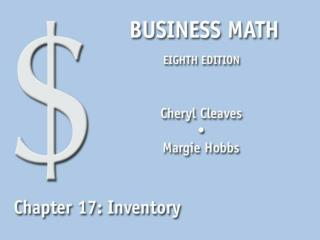17.1 Inventory

# 17.1 Inventory - PowerPoint PPT Presentation

17.1 Inventory. Use the following methods to find the ending inventory and cost of goods sold: Specific identification inventory Weighted average inventory method FIFO (first in, first out) LIFO (last in, first out) (continued on next slide). Inventory methods.I am the owner, or an agent authorized to act on behalf of the owner, of the copyrighted work described.
Download Presentation## 17.1 Inventory

An Image/Link below is provided (as is) to download presentation

Download Policy: Content on the Website is provided to you AS IS for your information and personal use and may not be sold / licensed / shared on other websites without getting consent from its author.While downloading, if for some reason you are not able to download a presentation, the publisher may have deleted the file from their server.

- - - - - - - - - - - - - - - - - - - - - - - - - - E N D - - - - - - - - - - - - - - - - - - - - - - - - - -
Presentation Transcript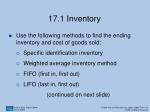17.1 Inventory
• Use the following methods to find the ending inventory and cost of goods sold:
• Specific identification inventory
• Weighted average inventory method
• FIFO (first in, first out)
• LIFO (last in, first out)

(continued on next slide)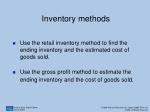Inventory methods
• Use the retail inventory method to find the ending inventory and the estimated cost of goods sold.
• Use the gross profit method to estimate the ending inventory and the cost of goods sold.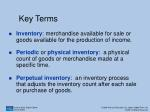Key Terms
• Inventory: merchandise available for sale or goods available for the production of income.
• Periodic or physical inventory: a physical count of goods or merchandise made at a specific time.
• Perpetual inventory: an inventory process that adjusts the inventory count after each sale or purchase of goods.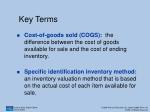Key Terms
• Cost-of-goods sold (COGS): the difference between the cost of goods available for sale and the cost of ending inventory.
• Specific identification inventory method: an inventory valuation method that is based on the actual cost of each item available for sale.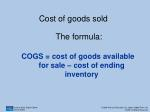Cost of goods sold

The formula:

COGS = cost of goods available for sale – cost of ending inventory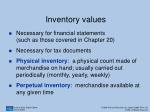Inventory values
• Necessary for financial statements (such as those covered in Chapter 20)
• Necessary for tax documents
• Physical inventory: a physical count made of merchandise on hand; usually carried out periodically (monthly, yearly, weekly)
• Perpetual inventory: merchandise available at any given time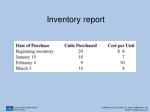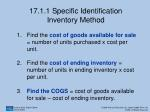17.1.1 Specific Identification Inventory Method
• Find the cost of goods available for sale= number of units purchased x cost per unit.
• Find the cost of ending inventory = number of units in ending inventory x cost per unit.

3. Find the COGS = cost of goods available for sale – cost of ending inventory.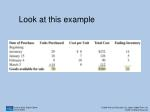Find the COGS
• Find the ending inventory by multiplying the number of items still available by the unit cost for the individual item.14 items x \$8 = \$112 5 items x \$7 = \$ 35 3 items x \$10 =\$ 30
• Add the cost of cost of goods available for sale and subtract that amount from the total cost. (\$560 - \$177 = \$383)
• The COGS = \$383Try this example
• Using Table 17-2, assume an ending inventory of 10 items for the first unit; 10 items for the second unit, 5 items for the third unit, and 2 units for the fourth item. Find the cost of ending inventory and COGS. (The total cost would be the same.)
• Cost of ending inventory = \$216
• COGS = \$34417.1.2 Use the Weighted-Average Method
• Cost of goods available for sale is divided by the number of units available for sale to get the average unit cost.
• Takes less time than finding exact prices for each unit.
• Used with goods that are similar in cost and have a relatively stable cost.How to use the weighted-average method
• Find the cost of goods available for sale = number of units purchased x cost per unit
• Find the average cost = cost of goods available for sale number of units available for sale
• Find the cost of ending inventory =number of units in ending inventory x average unit cost
• COGS = cost of goods available for sale – cost of ending inventoryLook at this example
• Refer to Table 17-2 on Slide 10 (or in the text).
• The average unit cost is the total cost of goods available for sale (\$560) divided by the number of units of goods available for sale (70) = \$8.
• Cost of ending inventory = cost of available goods (22) x average unit cost (\$8) = \$176.
• COGS = Total Cost – Cost of ending inventory
• COGS = \$560 - \$176 = \$384Try this example
• Refer to Slide 12 to calculate the total number of goods in ending inventory. (27 items)
• The average cost would be the same as the original example since only ending inventory amounts were modified. (\$8)
• Calculate the cost of ending inventory 27 items x \$8 = \$216
• COGS = TC (\$560) – cost of ending inventory (\$216) = \$34417.1.3 Use the FIFO Method
• FIFO = First in, first out
• Used by companies who want inventory costs to match replacement costs as closely as possible.
• The earliest units purchased are assumed to be the first units sold (first out).
• Cost of goods available for sale is relatively close to the current cost of purchasing additional items.Find the COGS using FIFO
• Find the cost of goods available for sale= number of units purchased x cost per unit.
• Find the assigned cost per unit by assuming those available in inventory are the latest ones purchased.
• Find the cost of ending inventory = number of units in inventory x assigned cost per unit.
• Find the COGS = cost of goods available for sale – cost of ending inventory.Look at this example
• Using Table 17-2 (in the text), find the COGS by assigning the cost of the latest items purchased.
• There are 22 units in the ending inventory and by using this method, they must be the latest units purchased.
• Count back in the table from the most recently purchased units until you have 22 units.
• March 3: 14 units at \$8 per unit (22-14 = 8 units left to be assigned)Example (continued)
• The remaining 8 units would be assigned a value of \$10 per unit.
• 14 units x \$8 = \$112; 8 units x \$10 =\$80\$112 + \$80 = \$192 (total of 22 units)
• COGS = \$560 - \$192 = \$368
• Using the FIFO method of inventory, the cost of goods sold is \$368.17.1.4 Find the COGS Using LIFO
• In this method, the latest units purchased (the last in) are assumed to be the first units sold (the first out).
• The cost of the ending inventory is figured on the cost of the oldest stock.
• The difference between the cost of goods available for sale and the replacement costs for new goods could be significant.
• Short-term profit on goods sold would be less since the newer, higher-priced goods were sold first.How to use the LIFO method
• Find the cost of goods available for sale= number of units purchased x cost per unit.
• Find the assigned cost per unit by assigning a cost per unit in the ending inventory assuming these units were the earliest units purchased.
• Find the cost of ending inventory = number of units in ending inventory x assigned cost per unit.
• COGS= cost of goods available for sale – cost of ending inventoryLook at this example
• Using Table 17-2, find the COGS using the LIFO method.
• Assign a cost for each unit in the ending inventory assuming these units were the earliest units purchased.
• 22 items at \$8 each = \$176
• Cost of ending inventory = \$176
• COGS= cost of goods available for sale (\$560) – cost of ending inventory (\$176)
• COGS = \$38417.1.5 Use the Retail Inventory Method
• Sometimes, businesses do not make monthly or periodic inventories; instead they estimate the cost of inventory rather than count goods individually.
• One method used to estimate inventory is called the retail method.
• To use this method to find the COGS, you also need to know the dollar value of sales.Key Terms
• Retail method: a method for estimating the value of inventory that is based on the cost ratio of the cost of goods available for sale and the retail value of goods available for sale.
• Ending inventory at cost: the cost of the ending inventory.
• Ending inventory at retail: the retail value of the ending inventory.Use the Retail Inventory Method
• Find the cost of goods available for sale = number of units purchased x cost per unit
• Find the retail value of goods available for sale
• Find the cost ratio =cost of goods available for sale retail value of goods available for sale
• Find the ending inventory at retail = retail value of goods available for sale - salesRetail method (cont.)

5. Find the ending inventory at cost= ending inventory at retail x cost ratio

6. Find the COGS= cost of goods available for sale – ending inventory at cost

(or) COGS = dollar value of sales x cost ratioLook at this example

Use the information from Table 17- 2 and the retail information on the right to find:

- the cost of ending inventory

–the COGS using the retail methodExample (continued)
• According to Table 17-2, the cost of goods available for sale is \$560.
• Retail price = \$800
• Cost ratio = \$560 ÷ \$800 = 0.7
• Ending inventory at retail = \$800 - \$487 = \$313
• Ending inventory at cost = \$313 x 0.7 = \$219.10
• COGS = \$487 x 0.7 = \$340.90 or COGS = \$560 - \$219.10 = \$340.90
• The ending inventory = \$219.10 and COGS = \$340.9017.1.6 Use the Gross Profit Method
• Gross profit margin method: a method of estimating the value of inventory that is based on a constant gross profit margin rate and net sales.
• Assumes a company maintains the same gross profit level from year to year.
• This method is not used for annual financial statements or calculating income taxes. It is a method for estimating inventory.Use the gross profit method
• Find the goods available for sale= beginning inventory + net purchases
• Find the estimated cost of goods sold= net sales x the complement of percent of gross profit
• Find the estimated ending inventory= goods available for sale – estimated cost of goods soldLook at this example
• Using the inventory report in Table 17-1 and net sales of \$487 to estimate inventory with the Gross Profit Method if gross profit on sales is 28%.
• Beginning inventory = 29 units x \$8 = \$232
• Net purchases= 18 units x \$7 + 9 units x \$10 + 14 units x \$8 = \$328
• Cost of goods available for sale= \$232 + \$328 = \$560Example (continued)
• Estimated cost of goods sold = net sales x the complement of percent of the gross profit margin\$487 (0.72) = \$350.64
• Estimated ending inventory =cost of goods available for sale- estimated cost of goods\$560 - \$350.64 = \$209.3617.2 Turnover and Overhead
• Find the inventory turnover rate
• Find the department overhead based on sales or floor space17.2.1 Find the Inventory Turnover Rate
• Inventory turnover rate will depend on type of business; a restaurant would have a high rate of turnover, but a furniture store may have a low rate of turnover.
• Inventory turnover: the frequency with which the inventory is sold and replaced.Low turnover

May indicate some or all of the following:

• Too much capital (company’s money) is tied up in inventory.
• Customers are dissatisfied with merchandise choice, quality or price.
• Merchandise is not properly marketed.High turnover

May indicate some or all of the following:

• Inventory is too small for the demand; thus, resulting in a loss of sales because merchandise is “out of stock.”
• Merchandise is highly desirable.
• Merchandise prices may be significantly lower than the competition’s prices.Turnover rate at cost

1.Average inventory at cost =

Beginning inventory at cost + Ending inventory at cost2

2. Divide the COGS by the average inventory at cost

Turnover rate at cost = COGS Average inventory at costLook at this example
• Ann’s Dress Shop has September sales of: \$52,500
• Cost of inventory on Sept. 1: \$15,890
• Cost of inventory on Sept. 30: \$18,000
• Average inventory at cost =\$15,890 + \$18,000 ÷ 2 = \$16,990
• Turnover rate at cost = \$52,500 ÷ \$16,990 =Turnover rate at cost = 3.09 or 3 times (rounded)
• The average inventory at cost is \$16,990 and the rate at cost is three times.Turnover rate at retail

1. Average inventory at retail =

Beg. inventory at retail + Ending inventory at retail2

2. Divide the sales by the average inventory at retail:

Turnover rate at retail = sales Average inventory at retailTurnover rate at retail
• Hungarian restaurant net sales: \$32,000
• Retail price of inventory on June 1: \$7,000
• Retail price of inventory on June 30: \$9,000
• Average inventory at retail \$7,000 + \$9,000 ÷ 2 = \$8,000
• Turnover rate at retail \$32,000 ÷ \$8,000 = 4
• The turnover rate at retail is four times in the month of June.Inventory turnover ratio
• The ratio shows the number of times a business’s inventory has been sold during a specified period.
• An inventory turnover of 3:1 for one year means that the store sold three times the value of the average inventory during the year.
• Another way to say this is that the merchandise was sold and replaced three times during the year.17.2.2 Department Overhead Based on Sales or Floor Space
• Overhead: expenses required for the operation of a business, such as salaries, office supplies, taxes, rent or mortgages, insurance and maintenance of equipment.
• The ratio between overhead and sales can say much about a firm’s efficiency or inefficiency.
• Overhead, like turnover, is another factor that lending institutions use in making decisions about business loans.Find department overhead based on sales
• Find the total sales: add the sales of the individual departments
• Find the department sales rate = department sales total sales
• Department overhead = department sales rate x total overheadFind department overhead based on floor space
• Find the total floor space: add the square feet of the floor space in each department
• Find the department floor space rate =floor space in department total floor space
• Find the overhead assigned to each department by floor space =department floor space rate x total overhead